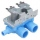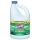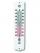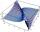# The pool

The pool has a volume of 40 m3 and the water temperature is 20 °C. How much water at 100 °C should we pour into the pool to increase the water temperature by 5 °C?

Result

x =  2.667 m3

#### Solution:

40*20+x*100 = (20+5)*(40+x)

75x = 200

x = 83 ≈ 2.666667

Calculated by our simple equation calculator.

Leave us a comment of example and its solution (i.e. if it is still somewhat unclear...):

Showing 0 comments:Be the first to comment!#### To solve this example are needed these knowledge from mathematics:

Looking for help with calculating arithmetic mean? Looking for help with calculating harmonic mean? Looking for a statistical calculator? Do you have a linear equation or system of equations and looking for its solution? Or do you have quadratic equation? Tip: Our volume units converter will help you with converion of volume units.

## Next similar examples:

1. The poolThe pool contains 220 m3 of water. The pool can be emptied either: a) 10 hours of pipe B and 8 hours of pipe A, or b) 10 hours of pipe A and 7 hours of pipe B. How many cubic meters of water will flow in 1 hour from pipe A and how many from pipe B?
2. Water temperaturesTo 25 liters of water at 50 °C we add 15 liters of water at a different temperature. How many °C should the water be colder than 50 °C so that 40 liters of the water obtained has a temperature 42.5 °C?
3. Mixing waterThe 30-liter container should we fill with water at 60 degrees Celsius. How many liters of water 80 degrees C hot and how many liters of water 20 degrees Celsius warm we have to mix?
4. Home cleaningMr. Smith is cleaning up a big mess at home. In the closet, he finds a solution that is 5% bleach, and another stronger solution that is 20% bleach. For this particular job, he needs 600mL of 15% bleach. How much of each type (to the nearest mL) should he.
5. BottlesThe must is sold in 5-liter and 2-liter bottles. Mr Kucera bought a total of 216 liters in 60 bottles. How many liters did Mr. Kucera buy in five-liter bottles?
6. Average temperatureThe average temperature from Monday to Sunday was 40.5 and the average temperature from Monday to Saturday was 42.8. What was the temperature on Sunday?
7. Daily temperatureThe average of daily temperature measurements in one week every day at the same hour was -2.8 °C. All temperatures were measured in different days are different. The highest daily maximum temperature was 2.4 °C, the lowest -6 °C. Determine the options tha
8. Median and modusRadka made 50 throws with a dice. The table saw fit individual dice's wall frequency: Wall Number: 1 2 3 4 5 6 frequency: 8 7 5 11 6 13 Calculate the modus and median of the wall numbers that Radka fell.
9. Theorem proveWe want to prove the sentense: If the natural number n is divisible by six, then n is divisible by three. From what assumption we started?
10. 75th percentile (quartille Q3)Find 75th percentile for 30,42,42,46,46,46,50,50,54
11. Harmonic and arithmetic meansThe local Utah Department of Child Service office wants to project staffing needs based on current social worker assignments. They have the number of cases per social worker for the following staff: Mary: 25 John: 35 Ted: 15 Lisa: 45 Anna: 20 Calculat
12. AverageIf the average(arithmetic mean) of three numbers x,y,z is 50. What is the average of there numbers (3x +10), (3y +10), (3z+10) ?
13. Meanif the mean of the set of data 5, 17, 19, 14, 15, 17, 7, 11, 16, 19, 5, 5, 10, 8, 13, 14, 4, 2, 17, 11, x is -91.74, what is the value of x?
14. Centre of massThe vertices of triangle ABC are from the line p distances 3 cm, 4 cm and 8 cm. Calculate distance from the center of gravity of the triangle to line p.
15. EquationHow many real roots has equation ? ?
16. CarpentersCarpenters 1 and 2 spend 10 days and 5 days respectively to make one table. If 50 tables were made by the first carpenter and 30 tables were made by the second carpenter, What is the average time spent on the products?
17. ChildrenThe group has 42 children. There are 4 more boys than girls. How many boys and girls are in the group?